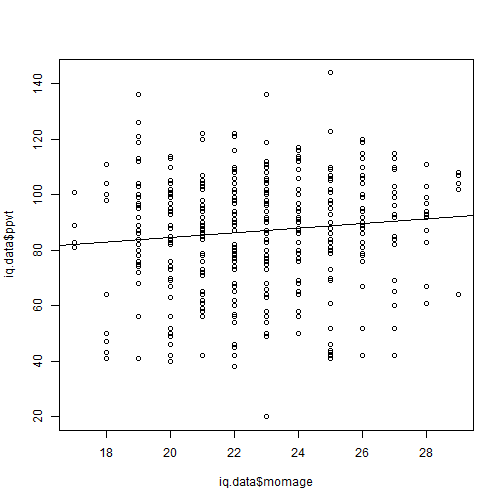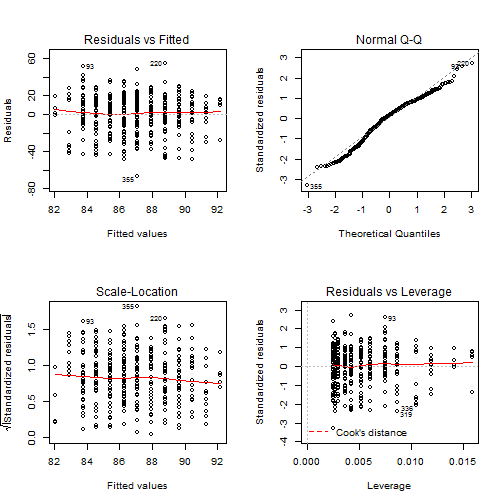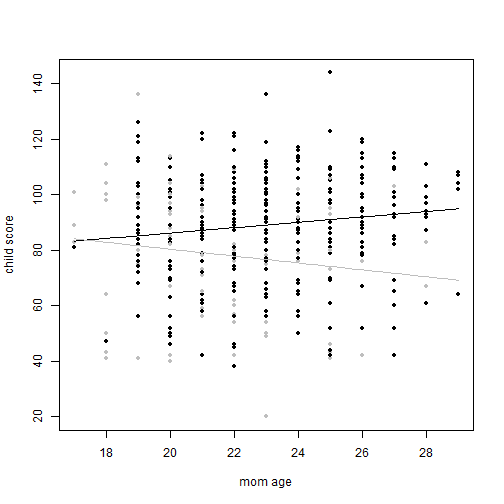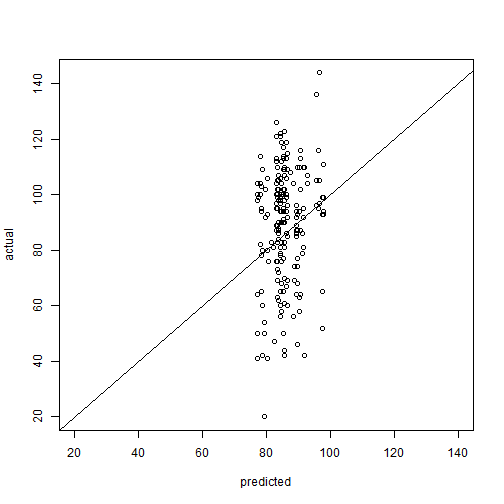# Chapter 3, Problem 4 (Gelman, Hill)

The child.iq folder contains a subset of the children and mother data discussed earlier in chapter 3. You have access to children's test scores at age 3, mother's education, and the mother's age at the time she gave birth for a sample of 400 children. The data are a Stata file which you can read into R as follows:

``````library(foreign)
attach(iq.data)
``````

The book doesn't tell you this, but mother's education is a categorical variable where 1 = no hs education, 2 = hs grad, 3 = some college, and 4 = college grad. I found this out here. It appears from the problem that the authors want us to treat this categorical variable as an integer value. If we wanted we could convert the variable to a factor as follows:

iq.data\$educ_cat <- as.factor(iq.data\$educ_cat)

Anyway, on to the exercises. The book has its own R library called “arm”. We'll load that so we can use its display() function. This provides a summary of a linear model that is cleaner and easier to read than the default R summary.

``````library(arm, quietly = TRUE, warn.conflicts = FALSE)
``````

part a
Fit a regression of child test scores on mother's age, display the data and fitted model, check assumptions, and interpret the slope coefficient. When do you recommend mothers should give birth? What are you assuming in making these recommendations?

``````fit.4 <- lm(ppvt ~ momage, data = iq.data)
display(fit.4)
``````
``````## lm(formula = ppvt ~ momage, data = iq.data)
##             coef.est coef.se
## (Intercept) 67.78     8.69
## momage       0.84     0.38
## ---
## n = 400, k = 2
## residual sd = 20.34, R-Squared = 0.01
``````
``````plot(iq.data\$momage, iq.data\$ppvt)
abline(fit.4)
````````````par(mfrow = c(2, 2))
plot(fit.4)
``````When should mothes give birth? According to this model, in their late 20s. I'm assuming the model is valid and that mother's education has no effect. I'm guessing this won't be the case as we continue with the exercise.

part b
Repeat this for a regression that further includes mother's education, interpreting both slope coefficients in this model. Have your conclusions about the timing of birth changed?

This is why I think the authors want us to treat education level as an integer. The say “both” slope coefficients. If we converted to factors, there would be four slope coefficients: one for age and three for the levels (with the first level serving as baseline).

``````fit.4b <- lm(ppvt ~ momage + educ_cat, data = iq.data)
display(fit.4b)
``````
``````## lm(formula = ppvt ~ momage + educ_cat, data = iq.data)
##             coef.est coef.se
## (Intercept) 69.16     8.57
## momage       0.34     0.40
## educ_cat     4.71     1.32
## ---
## n = 400, k = 3
## residual sd = 20.05, R-Squared = 0.04
``````

Indeed my conclusions have changed. Education appears to be a significant predictor of test score.

part c
Now create an indicator variable reflecting whether the mother has completed high school or not. Consider interactions between the high school completion and mother's age in family. Also, create a plot that shows the separate regression lines for each high school completion status group.

``````# create indicator for hs education or not
iq.data\$hs <- ifelse(iq.data\$educ_cat >= 2, 1, 0)

fit.4c <- lm(ppvt ~ hs * momage, data = iq.data)
display(fit.4c)
``````
``````## lm(formula = ppvt ~ hs * momage, data = iq.data)
##             coef.est coef.se
## (Intercept) 105.22    17.65
## hs          -38.41    20.28
## momage       -1.24     0.81
## hs:momage     2.21     0.92
## ---
## n = 400, k = 4
## residual sd = 19.85, R-Squared = 0.06
``````
``````
colors <- ifelse(iq.data\$hs == 1, "black", "gray")
plot(momage, ppvt, xlab = "mom age", ylab = "child score", col = colors, pch = 20)
curve(cbind(1, 1, x, 1 * x) %*% coef(fit.4c), add = TRUE, col = "black")  #finished hs
curve(cbind(1, 0, x, 0 * x) %*% coef(fit.4c), add = TRUE, col = "gray")  # not finish hs
``````part d
Finally, fit a regression of child test scores on mother's age and education level for the first 200 children and use this model to predict test scores for the next 200. Graphically display comparisons of the predicted and actual scores for the final 200 children.

``````fit.4d <- lm(ppvt ~ momage + educ_cat, data = iq.data[1:200, ])
display(fit.4d)
``````
``````## lm(formula = ppvt ~ momage + educ_cat, data = iq.data[1:200,
##     ])
##             coef.est coef.se
## (Intercept) 63.63    11.82
## momage       0.45     0.55
## educ_cat     5.44     1.82
## ---
## n = 200, k = 3
## residual sd = 19.58, R-Squared = 0.06
``````
``````pred.4d <- predict(fit.4d, iq.data[201:400, ])

# display comparison of predicted and actual scores for final 200 kids
plot(pred.4d, iq.data\$ppvt[201:400], xlim = c(20, 140), xlab = "predicted",
ylab = "actual")
abline(a = 0, b = 1)
``````